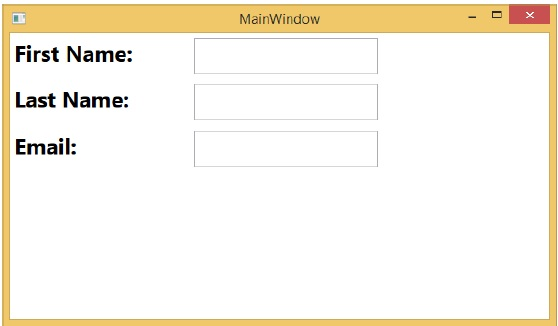# WPF - Window Level

Defining a style on a window level can make it accessible by all the elements on that window. Given below is an example of window level where all the three text blocks have a common style.

```<Window x:Class = "Styles.MainWindow"
xmlns = "http://schemas.microsoft.com/winfx/2006/xaml/presentation"
xmlns:x = "http://schemas.microsoft.com/winfx/2006/xaml"
Title = "MainWindow" Height = "350" Width = "604">

<Window.Resources>
<Style TargetType = "TextBlock">
<Setter Property = "FontSize" Value = "24" />
<Setter Property = "Margin" Value = "5" />
<Setter Property = "FontWeight" Value = "Bold" />
</Style>

<Style TargetType = "TextBox">
<Setter Property = "HorizontalAlignment" Value = "Left" />
<Setter Property = "FontSize" Value = "24" />
<Setter Property = "Margin" Value = "5" />
<Setter Property = "Width" Value = "200" />
<Setter Property = "Height" Value = "40" />
</Style>
</Window.Resources>

<Grid>
<Grid.RowDefinitions>
<RowDefinition Height = "Auto" />
<RowDefinition Height = "Auto" />
<RowDefinition Height = "Auto" />
<RowDefinition Height = "*" />
</Grid.RowDefinitions>

<Grid.ColumnDefinitions>
<ColumnDefinition Width = "*" />
<ColumnDefinition Width = "2*" />
</Grid.ColumnDefinitions>

<TextBlock Text = "First Name: "/>
<TextBox Name = "FirstName" Grid.Column = "1" />
<TextBlock Text = "Last Name: " Grid.Row = "1" />
<TextBox Name = "LastName" Grid.Column = "1" Grid.Row = "1" />
<TextBlock Text = "Email: " Grid.Row = "2" />
<TextBox Name = "Email" Grid.Column = "1" Grid.Row = "2"/>
</Grid>

</Window>
```

When you compile and execute the above code, it will produce the following window.wpf_styles.htm